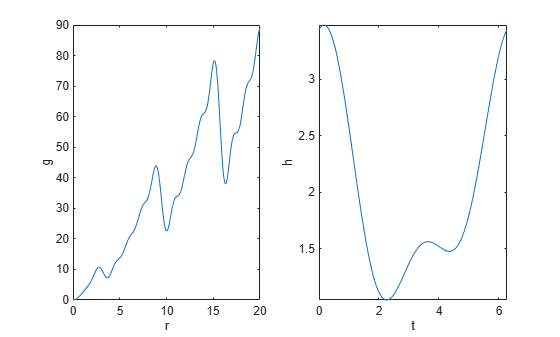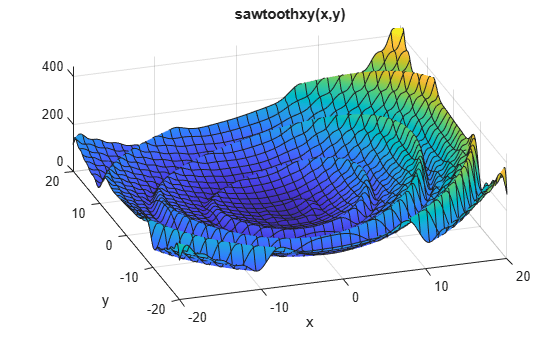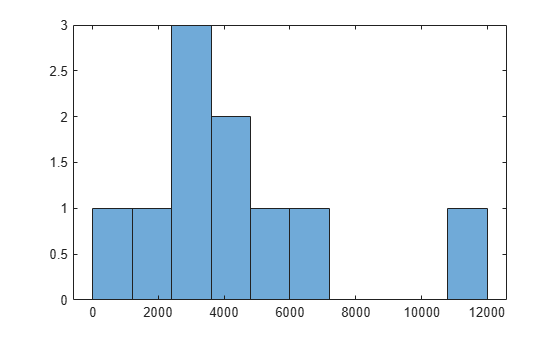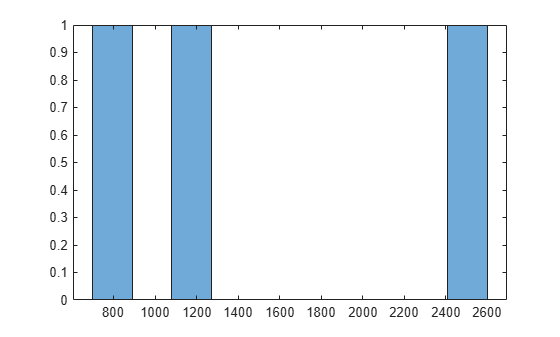# Find Global or Multiple Local Minima

This example illustrates how `GlobalSearch` finds a global minimum efficiently, and how `MultiStart` finds many more local minima.

The objective function for this example has many local minima and a unique global minimum. In polar coordinates, the function is

`$f\left(r,t\right)=g\left(r\right)h\left(t\right)$`

where

`$\begin{array}{l}g\left(r\right)=\left(\mathrm{sin}\left(r\right)-\frac{\mathrm{sin}\left(2r\right)}{2}+\frac{\mathrm{sin}\left(3r\right)}{3}-\frac{\mathrm{sin}\left(4r\right)}{4}+4\right)\frac{{r}^{2}}{r+1}\\ h\left(t\right)=2+\mathrm{cos}\left(t\right)+\frac{\mathrm{cos}\left(2t-\frac{1}{2}\right)}{2}.\end{array}$`

Plot the functions $g$ and $h$, and create a surface plot of the function $f$.

```figure subplot(1,2,1); fplot(@(r)(sin(r) - sin(2*r)/2 + sin(3*r)/3 - sin(4*r)/4 + 4) .* r.^2./(r+1), [0 20]) title(''); ylabel('g'); xlabel('r'); subplot(1,2,2); fplot(@(t)2 + cos(t) + cos(2*t-1/2)/2, [0 2*pi]) title(''); ylabel('h'); xlabel('t');``````figure fsurf(@(x,y) sawtoothxy(x,y), [-20 20]) % sawtoothxy is defined in the first step below xlabel('x'); ylabel('y'); title('sawtoothxy(x,y)'); view(-18,52)```The global minimum is at , with objective function 0. The function $g\left(r\right)$ grows approximately linearly in $r$, with a repeating sawtooth shape. The function $h\left(t\right)$ has two local minima, one of which is global.

The `sawtoothxy.m` file converts from Cartesian to polar coordinates, then computes the value in polar coordinates.

`type sawtoothxy`
```function f = sawtoothxy(x,y) [t r] = cart2pol(x,y); % change to polar coordinates h = cos(2*t - 1/2)/2 + cos(t) + 2; g = (sin(r) - sin(2*r)/2 + sin(3*r)/3 - sin(4*r)/4 + 4) ... .*r.^2./(r+1); f = g.*h; end ```

### Single Global Minimum Via `GlobalSearch`

To search for the global minimum using `GlobalSearch`, first create a problem structure. Use the `'sqp'` algorithm for `fmincon`,

```problem = createOptimProblem('fmincon',... 'objective',@(x)sawtoothxy(x(1),x(2)),... 'x0',[100,-50],'options',... optimoptions(@fmincon,'Algorithm','sqp','Display','off'));```

The start point is `[100,-50]` instead of `[0,0]` so `GlobalSearch` does not start at the global solution.

Validate the problem structure by running `fmincon`.

`[x,fval] = fmincon(problem)`
```x = 1×2 45.7236 -107.6515 ```
```fval = 555.5820 ```

Create the `GlobalSearch` object, and set iterative display.

`gs = GlobalSearch('Display','iter');`

For reproducibility, set the random number generator seed.

`rng(14,'twister')`

Run the solver.

`[x,fval] = run(gs,problem)`
``` Num Pts Best Current Threshold Local Local Analyzed F-count f(x) Penalty Penalty f(x) exitflag Procedure 0 200 555.6 555.6 0 Initial Point 200 1463 1.547e-15 1.547e-15 1 Stage 1 Local 300 1564 1.547e-15 5.858e+04 1.074 Stage 2 Search 400 1664 1.547e-15 1.84e+05 4.16 Stage 2 Search 500 1764 1.547e-15 2.683e+04 11.84 Stage 2 Search 600 1864 1.547e-15 1.122e+04 30.95 Stage 2 Search 700 1964 1.547e-15 1.353e+04 65.25 Stage 2 Search 800 2064 1.547e-15 6.249e+04 163.8 Stage 2 Search 900 2164 1.547e-15 4.119e+04 409.2 Stage 2 Search 950 2359 1.547e-15 477 589.7 387 2 Stage 2 Local 952 2423 1.547e-15 368.4 477 250.7 2 Stage 2 Local 1000 2471 1.547e-15 4.031e+04 530.9 Stage 2 Search GlobalSearch stopped because it analyzed all the trial points. 3 out of 4 local solver runs converged with a positive local solver exit flag. ```
```x = 1×2 10-7 × 0.0414 0.1298 ```
```fval = 1.5467e-15 ```

The solver finds three local minima, including the global minimum near [0,0].

### Multiple Local Minima Via `MultiStart`

To search for multiple minima using `MultiStart`, first create a problem structure. Because the problem is unconstrained, use the `fminunc` solver. Set options not to show any display at the command line.

```problem = createOptimProblem('fminunc',... 'objective',@(x)sawtoothxy(x(1),x(2)),... 'x0',[100,-50],'options',... optimoptions(@fminunc,'Display','off'));```

Validate the problem structure by running it.

`[x,fval] = fminunc(problem)`
```x = 1×2 8.4420 -110.2602 ```
```fval = 435.2573 ```

Create a default `MultiStart` object.

`ms = MultiStart;`

Run the solver for 50 iterations, recording the local minima.

```rng(1) % For reproducibility [x,fval,eflag,output,manymins] = run(ms,problem,50)```
```MultiStart completed some of the runs from the start points. 10 out of 50 local solver runs converged with a positive local solver exitflag. ```
```x = 1×2 -73.8348 -197.7810 ```
```fval = 766.8260 ```
```eflag = 2 ```
```output = struct with fields: funcCount: 8583 localSolverTotal: 50 localSolverSuccess: 10 localSolverIncomplete: 40 localSolverNoSolution: 0 message: 'MultiStart completed some of the runs from the start points. ...' ```
```manymins=1×10 object 1x10 GlobalOptimSolution array with properties: X Fval Exitflag Output X0 ```

The solver does not find the global minimum near `[0,0]`. The solver finds 10 distinct local minima.

Plot the function values at the local minima:

`histogram([manymins.Fval],10)`Plot the function values at the three best points:

```bestf = [manymins.Fval]; histogram(bestf(1:3),10)````MultiStart` starts `fminunc` from start points with components uniformly distributed between –1000 and 1000. `fminunc` often gets stuck in one of the many local minima. `fminunc` exceeds its iteration limit or function evaluation limit 40 times.